Chapter 12 – Wave Optics

# 12.3 Young’s Double Slit Experiment

### λSummary

• Explain the phenomena of interference.
• Define constructive interference for a double slit and destructive interference for a double slit.

Although Christiaan Huygens thought that light was a wave, Isaac Newton did not. Newton felt that there were other explanations for colour, and for the interference and diffraction effects that were observable at the time. Owing to Newton’s tremendous stature, his view generally prevailed. The fact that Huygens’s principle worked was not considered evidence that was direct enough to prove that light is a wave. The acceptance of the wave character of light came many years later when, in 1801, the English physicist and physician Thomas Young (1773–1829) did his now-classic double slit experiment (see Figure 1).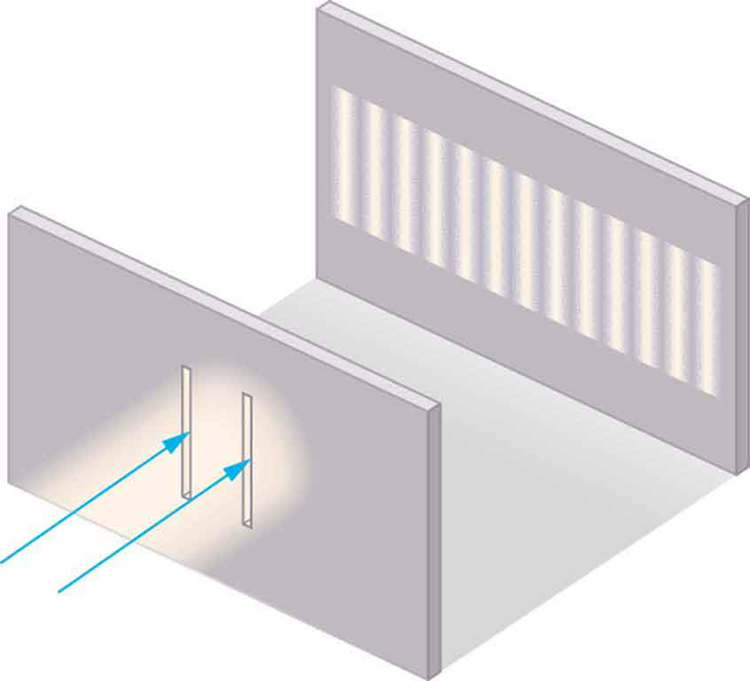Figure 1. Young’s double slit experiment. Here pure-wavelength light sent through a pair of vertical slits is diffracted into a pattern on the screen of numerous vertical lines spread out horizontally. Without diffraction and interference, the light would simply make two lines on the screen.

Why do we not ordinarily observe wave behaviour for light, such as observed in Young’s double slit experiment? First, light must interact with something small, such as the closely spaced slits used by Young, to show pronounced wave effects. Furthermore, Young first passed light from a single source (the Sun) through a single slit to make the light somewhat coherent. By coherent, we mean waves are in phase or have a definite phase relationship. Incoherent means the waves have random phase relationships. Why did Young then pass the light through a double slit? The answer to this question is that two slits provide two coherent light sources that then interfere constructively or destructively. Young used sunlight, where each wavelength forms its own pattern, making the effect more difficult to see. We illustrate the double slit experiment with monochromatic (single colour, single wavelength,  λ) light to clarify the effect. Figure 2 shows the pure constructive and destructive interference of two waves having the same wavelength and amplitude.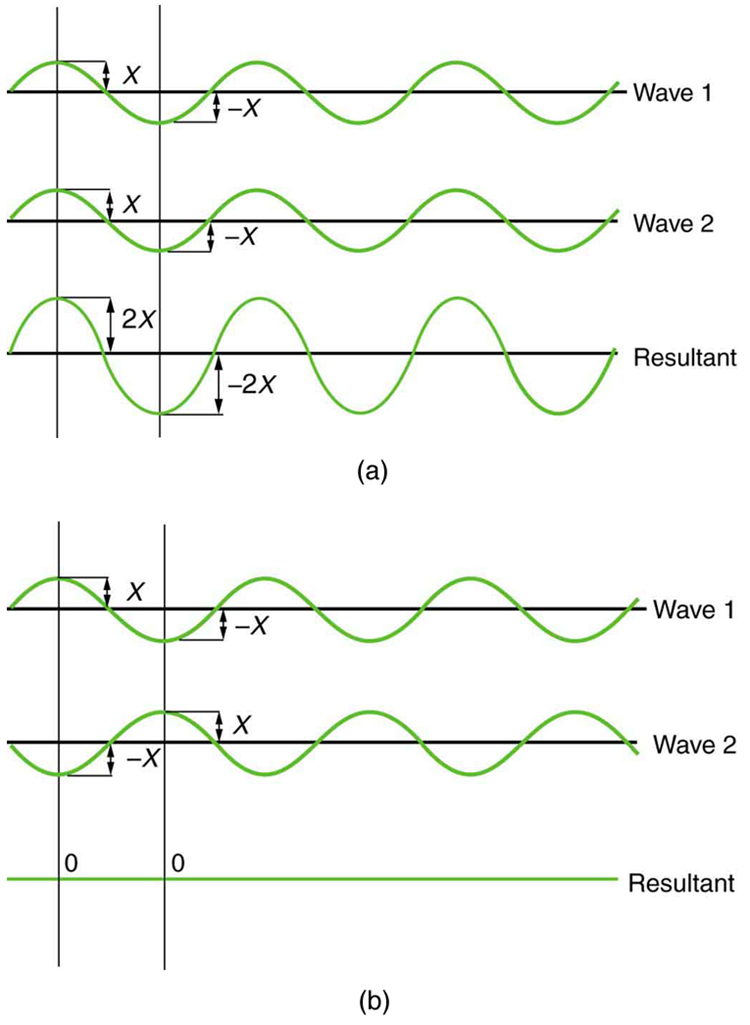Figure 2. The amplitudes of waves add. (a) Pure constructive interference is obtained when identical waves are in phase. (b) Pure destructive interference occurs when identical waves are exactly out of phase, or shifted by half a wavelength.

When light passes through narrow slits, it is diffracted into semicircular waves, as shown in Figure 3(a). Pure constructive interference occurs where the waves are crest to crest or trough to trough. Pure destructive interference occurs where they are crest to trough. The light must fall on a screen and be scattered into our eyes for us to see the pattern. An analogous pattern for water waves is shown in Figure 3(b). Note that regions of constructive and destructive interference move out from the slits at well-defined angles to the original beam. These angles depend on wavelength and the distance between the slits, as we shall see below.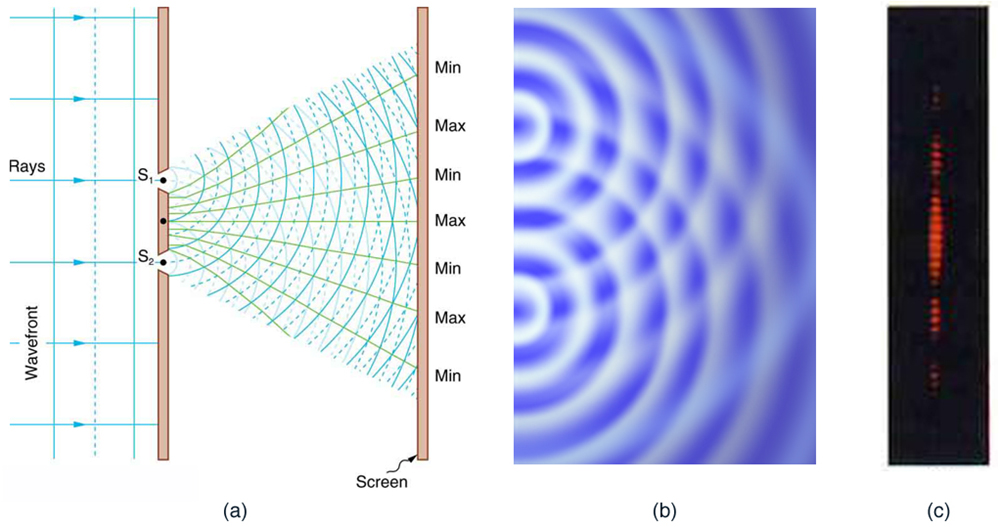Figure 3. Double slits produce two coherent sources of waves that interfere. (a) Light spreads out (diffracts) from each slit, because the slits are narrow. These waves overlap and interfere constructively (bright lines) and destructively (dark regions). We can only see this if the light falls onto a screen and is scattered into our eyes. (b) Double slit interference pattern for water waves are nearly identical to that for light. Wave action is greatest in regions of constructive interference and least in regions of destructive interference. (c) When light that has passed through double slits falls on a screen, we see a pattern such as this. (credit: PASCO)

To understand the double slit interference pattern, we consider how two waves travel from the slits to the screen, as illustrated in Figure 4. Each slit is a different distance from a given point on the screen. Thus different numbers of wavelengths fit into each path. Waves start out from the slits in phase (crest to crest), but they may end up out of phase (crest to trough) at the screen if the paths differ in length by half a wavelength, interfering destructively as shown in Figure 4(a). If the paths differ by a whole wavelength, then the waves arrive in phase (crest to crest) at the screen, interfering constructively as shown in Figure 4(b). More generally, if the paths taken by the two waves differ by any half-integral number of wavelengths (1/2λ, 3/2 λ, 5/2 λ …  etc.) then destructive interference occurs. Similarly, if the paths taken by the two waves differ by any integral number of wavelengths (1λ, 2λ, 3λ… , etc.), then constructive interference occurs.

### Take-Home Experiment: Using Fingers as Slits

Look at a light, such as a street lamp or incandescent bulb, through the narrow gap between two fingers held close together. What type of pattern do you see? How does it change when you allow the fingers to move a little farther apart? Is it more distinct for a monochromatic source, such as the yellow light from a sodium vapour lamp, than for an incandescent bulb?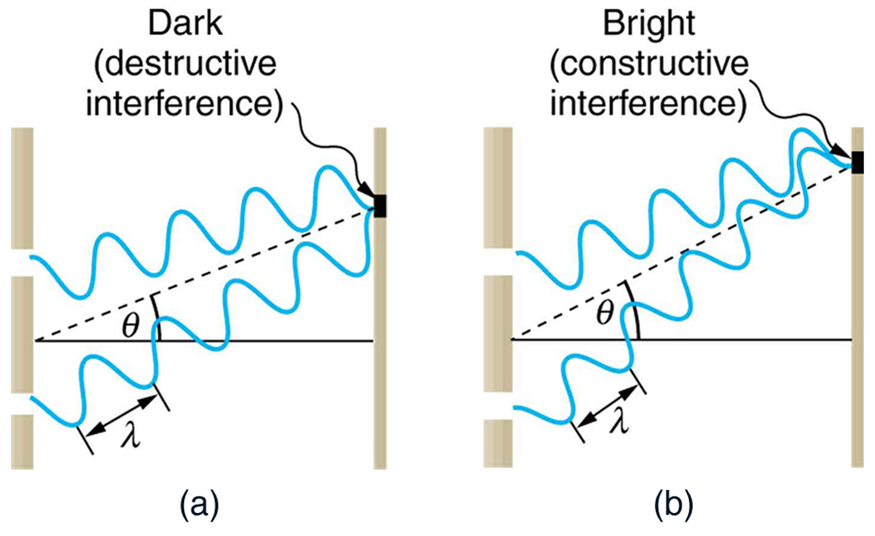Figure 4. Waves follow different paths from the slits to a common point on a screen. (a) Destructive interference occurs here, because one path is a half wavelength longer than the other. The waves start in phase but arrive out of phase. (b) Constructive interference occurs here because one path is a whole wavelength longer than the other. The waves start out and arrive in phase.
PhET simulation.  Direct link:  https://phet.colorado.edu/sims/html/wave-interference/latest/wave-interference_en.html .  If you are reading this online then it will open directly below.

Figure 5 below shows how to determine the path length difference for waves traveling from two slits to a common point on a screen. If the screen is a large distance away compared with the distance between the slits, then the angle θ between the path and a line from the slits to the screen (see the figure) is nearly the same for each path. The difference between the paths is shown in the figure; simple trigonometry shows it to be dsinθ, where d is the distance between the slits. To obtain constructive interference for a double slit, the path length difference must be an integral multiple of the wavelength, or

d sinθ = mλ  for  m = 0,  1, -1, 2, -2, 3, -3, 4, -4, ….(constructive)

Similarly, to obtain destructive interference for a double slit, the path length difference must be a half-integral multiple of the wavelength, or

d sinθ = (m  + 1/2 λ)  for  m = 0,  1, -1, 2, -2, 3, -3, 4, -4, ….(destructive)

where λ is the wavelength of the light, d is the distance between slits, and θ is the angle from the original direction of the beam as discussed above. We call m the order of the interference. For example, m=4 is fourth-order interference.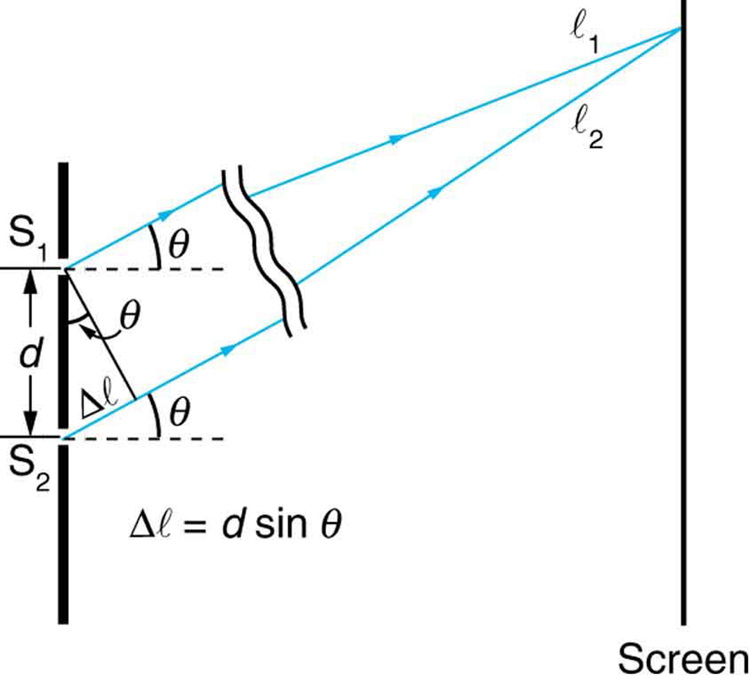Figure 5. The paths from each slit to a common point on the screen differ by an amount d sin θ, assuming the distance to the screen is much greater than the distance between slits (not to scale here).

The equations for double slit interference imply that a series of bright and dark lines are formed. For vertical slits, the light spreads out horizontally on either side of the incident beam into a pattern called interference fringes, illustrated in Figure 6. The intensity of the bright fringes falls off on either side, being brightest at the centre. The closer the slits are, the more is the spreading of the bright fringes. We can see this by examining the equation

d sinθ = mλ  for  m = 0,  1, -1, 2, -2, 3, -3, 4, -4, ….(constructive)

For fixed  λ  and m, the smaller d is, the larger θ must be, since dsinθ= mλ.

This is consistent with our contention that wave effects are most noticeable when the object the wave encounters (here, slits a distance d apart) is small. Small d gives large θ, hence a large effect.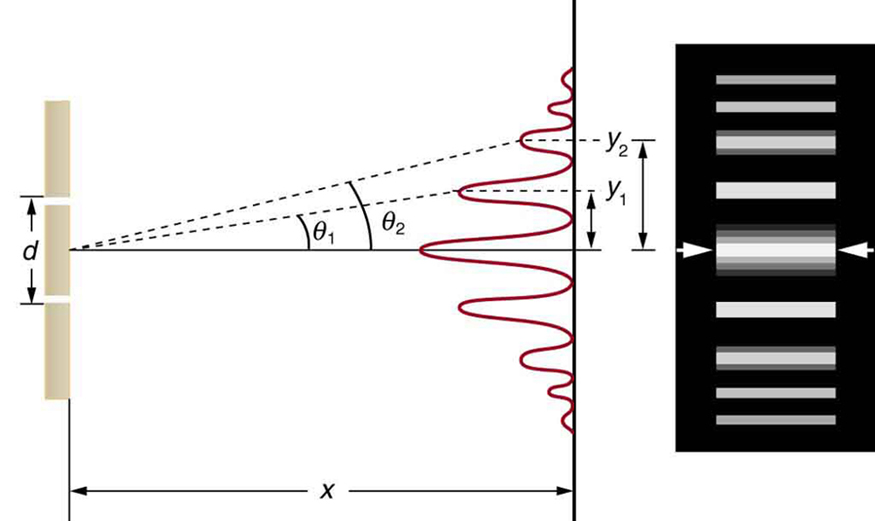Figure 6. The interference pattern for a double slit has an intensity that falls off with angle. The photograph shows multiple bright and dark lines, or fringes, formed by light passing through a double slit.

### Example 1: Finding a Wavelength from an Interference Pattern

Suppose you pass light from a He-Ne laser through two slits separated by 0.0100 mm and find that the third bright line on a screen is formed at an angle of 10.95o relative to the incident beam. What is the wavelength of the light?

Strategy

The third bright line is due to third-order constructive interference, which means that m=3. We are given d = 0.0100 mm and θ = 10.95o. The wavelength can thus be found using the equation d sinθ = m λ for constructive interference.

Solution

The equation is d sinθ = m λ. Solving for the wavelength λ gives

λ = (d sinθ)  / m

Substituting known values yields

λ = (d sinθ) / m  = (0.0100 x 10-3 m) (sin 10.95o)  /  3 = 6.33 x 10-7 m  or

λ  =  633 nm

Discussion

To three digits, this is the wavelength of light emitted by the common He-Ne laser. Not by coincidence, this red colour is similar to that emitted by neon lights. More important, however, is the fact that interference patterns can be used to measure wavelength. Young did this for visible wavelengths. This analytical technique is still widely used to measure electromagnetic spectra. For a given order, the angle for constructive interference increases with λ, so that spectra (measurements of intensity versus wavelength) can be obtained.

### Example 2: Calculating Highest Order Possible

Interference patterns do not have an infinite number of lines, since there is a limit to how big m can be. What is the highest-order constructive interference possible with the system described in the preceding example?

Strategy and Concept

The equation d sinθ = mλ  for  m = 0,  1, -1, 2, -2, 3, -3, 4, -4, … describes constructive interference. For fixed values of d and λ, the larger m is, the larger sinθ is. However, the maximum value that sinθ can have is 1, for an angle of 90o. (Larger angles imply that light goes backward and does not reach the screen at all.) Let us find which m corresponds to this maximum diffraction angle.

Solution

Solving the equation d sinθ = mλ  for m gives

m = (d sinθ) / λ

Taking sinθ =sin90o= 1 and substituting the values of d and λ, and remembering the values for the metric prefixes, from the preceding example gives

m = ( 0.0100 x 10-3 m ) (1) / (633 x10-9 m) ≈ 15.8

Therefore, the largest integer m can be is 15, or

m = 15.

Discussion

The number of fringes depends on the wavelength and slit separation. The number of fringes will be very large for large slit separations. However, if the slit separation becomes much greater than the wavelength, the intensity of the interference pattern changes so that the screen has two bright lines cast by the slits, as expected when light behaves like a ray. We also note that the fringes get fainter further away from the centre. Consequently, not all 15 fringes may be observable.

# Section Summary

• Young’s double slit experiment gave definitive proof of the wave character of light.
• An interference pattern is obtained by the superposition of light from two slits.
• There is constructive interference when d sinθ = mλ  for  m = 0, 1, -1, 2, -2, 3, -3, 4, -4, ….(constructive), where d is the distance between the slits, θ is the angle relative to the incident direction, and m is the order of the interference.
• There is destructive interference when d sinθ =  ( m + 1/2 )λ   for  m = 0, 1, -1, 2, -2, 3, -3, 4, -4, ….(constructive)

### Conceptual Questions

1: Young’s double slit experiment breaks a single light beam into two sources. Would the same pattern be obtained for two independent sources of light, such as the headlights of a distant car? Explain.

2: Suppose you use the same double slit to perform Young’s double slit experiment in air and then repeat the experiment in water. Do the angles to the same parts of the interference pattern get larger or smaller? Does the colour of the light change? Explain.

3: Is it possible to create a situation in which there is only destructive interference? Explain.

4: Figure 7 shows the central part of the interference pattern for a pure wavelength of red light projected onto a double slit. The pattern is actually a combination of single slit and double slit interference. Note that the bright spots are evenly spaced. Is this a double slit or single slit characteristic? Note that some of the bright spots are dim on either side of the centre. Is this a single slit or double slit characteristic? Which is smaller, the slit width or the separation between slits? Explain your responses.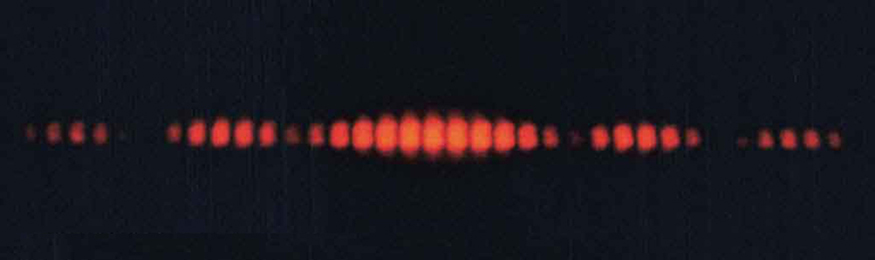Figure 7. This double slit interference pattern also shows signs of single slit interference. (credit: PASCO)

### Problems & Exercises

1. At what angle is the first-order maximum for 450-nm wavelength blue light falling on double slits separated by 0.0500 mm?
2. Calculate the angle for the third-order maximum of 580-nm wavelength yellow light falling on double slits separated by 0.100 mm.
3. What is the separation between two slits for which 610-nm orange light has its first maximum at an angle of 30.0 degrees?
4. Find the distance between two slits that produces the first minimum for 410-nm violet light at an angle of 45.0  degrees.
5.  Calculate the wavelength of light that has its third minimum at an angle of 30.0o when falling on double slits separated by 3.00 μm. Explicitly, show how you follow the steps in  Problem-Solving Strategies for Wave Optics.
6. What is the wavelength of light falling on double slits separated by 2.00 μm if the third-order maximum is at an angle of 60.0o?
7. At what angle is the fourth-order maximum for the situation in Problems & Exercises 1?
8. What is the highest-order maximum for 400-nm light falling on double slits separated by 25.0 μm?
9. Find the largest wavelength of light falling on double slits separated by 1.20 μm for which there is a first-order maximum. Is this in the visible part of the spectrum?
10. What is the smallest separation between two slits that will produce a second-order maximum for 720-nm red light?
11. (a) What is the smallest separation between two slits that will produce a second-order maximum for any visible light? (b) For all visible light.
12. (a) If the first-order maximum for pure-wavelength light falling on a double slit is at an angle of 10.0o, at what angle is the second-order maximum? (b) What is the angle of the first minimum? (c) What is the highest-order maximum possible here?
13. Figure 8 shows a double slit located a distance x from a screen, with the distance from the centre of the screen given by y.  When the distance d between the slits is relatively large, there will be numerous bright spots, called fringes. Show that, for small angles (where sinθ≈tanθ, with θ in radians), the distance between fringes is given by Δy = x λ /d.

14: Using the result of the problem above, calculate the distance between fringes for 633-nm light falling on double slits separated by 0.0800 mm, located 3.00 m from a screen as in Figure 8.

15: Using the result of the problem two problems prior, find the wavelength of light that produces fringes 7.50 mm apart on a screen 2.00 m from double slits separated by 0.120 mm (see Figure 8).

## Glossary

coherent
waves are in phase or have a definite phase relationship
constructive interference for a double slit
the path length difference must be an integral multiple of the wavelength
destructive interference for a double slit
the path length difference must be a half-integral multiple of the wavelength
incoherent
waves have random phase relationships
order
the integer m  used in the equations for constructive and destructive interference for a double slit

### Solutions

Problems & Exercises

1: 0.516o

2: 0.997o

3: 1.22 x10-6 m

4: 0.290 μm

5: 600 nm

6: 577 nm

7: 2.06o

8: m=62

9: 1200 nm (not visible)

10:  1.44 μm

11: (a) 760 nm (b) 1520 nm

12:  m=6

13: For small angles sinθ ≈ tanθ≈θ when the angle is in radians

For two adjacent fringes we have d sinθm = m λ

and d sinθm +1 =  (m+1) λ

Subtracting these equations gives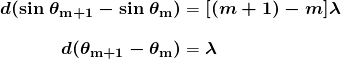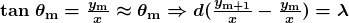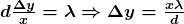14: 2.37 cm

15: 450 nm